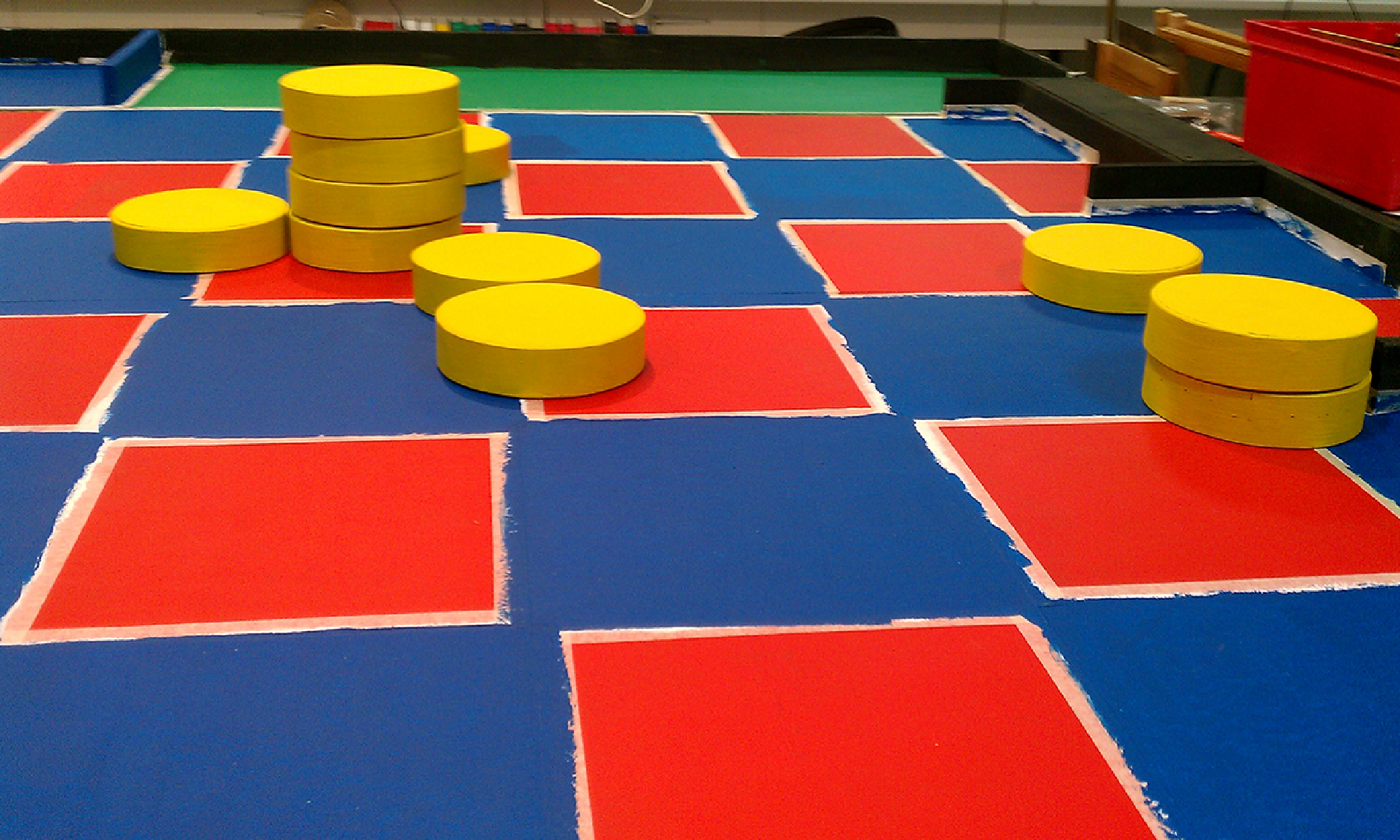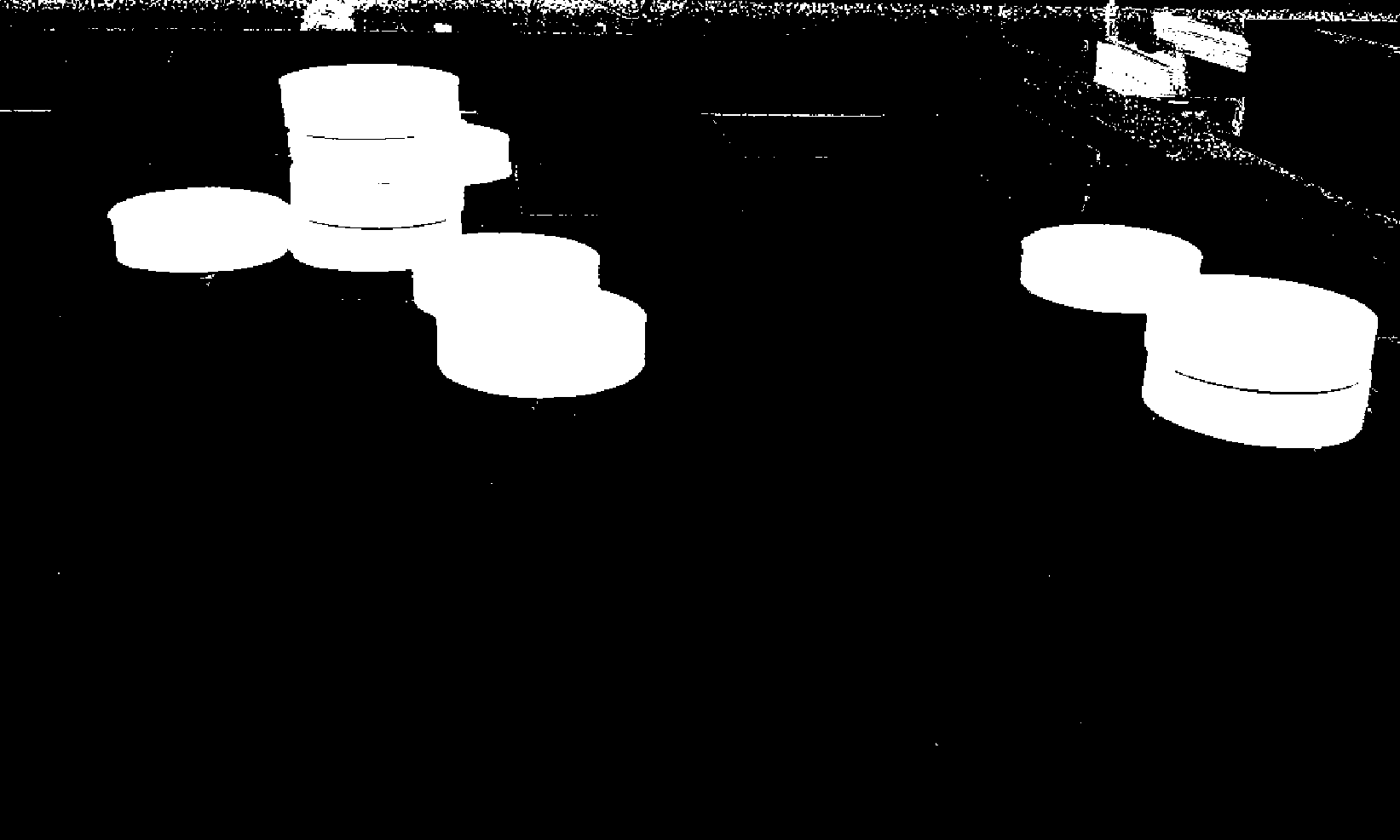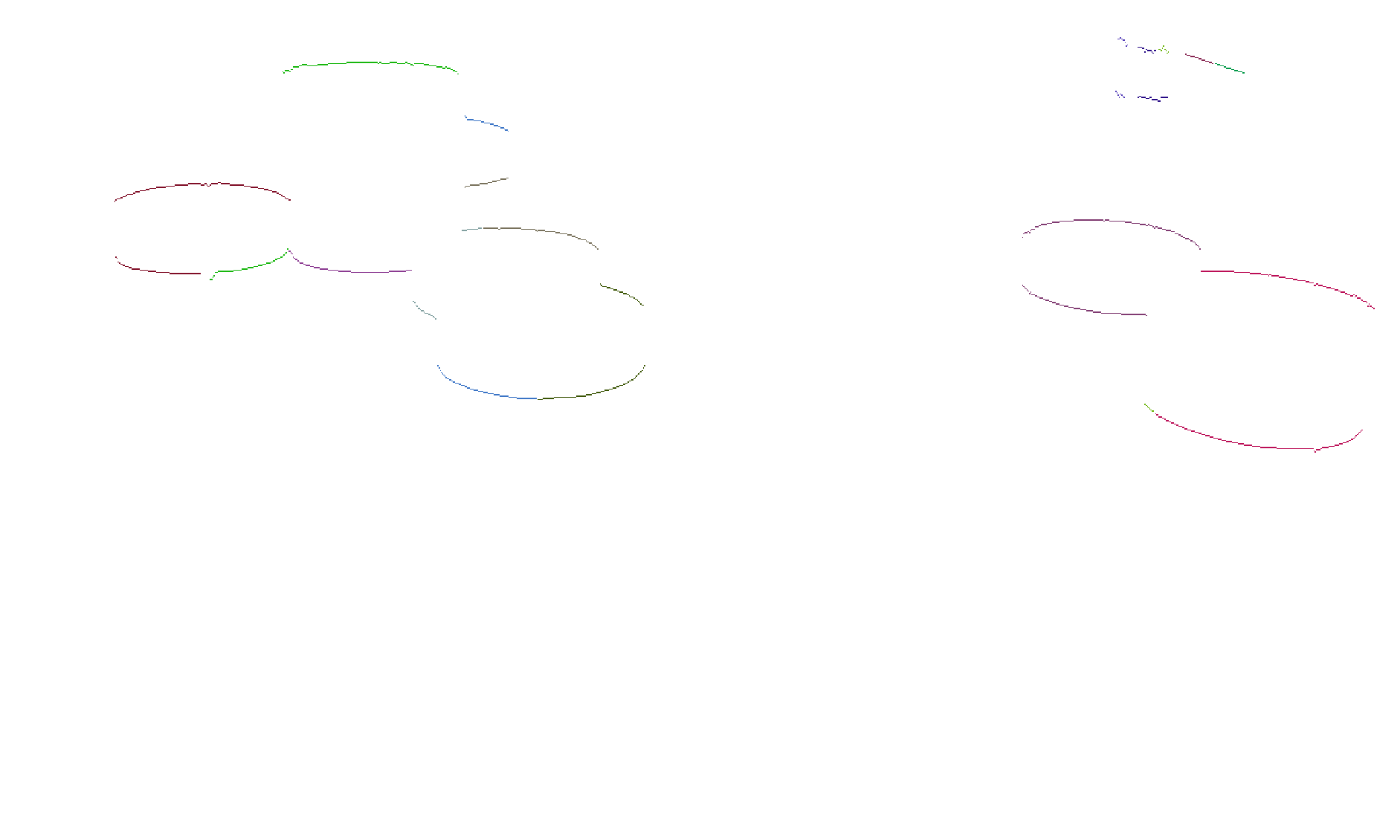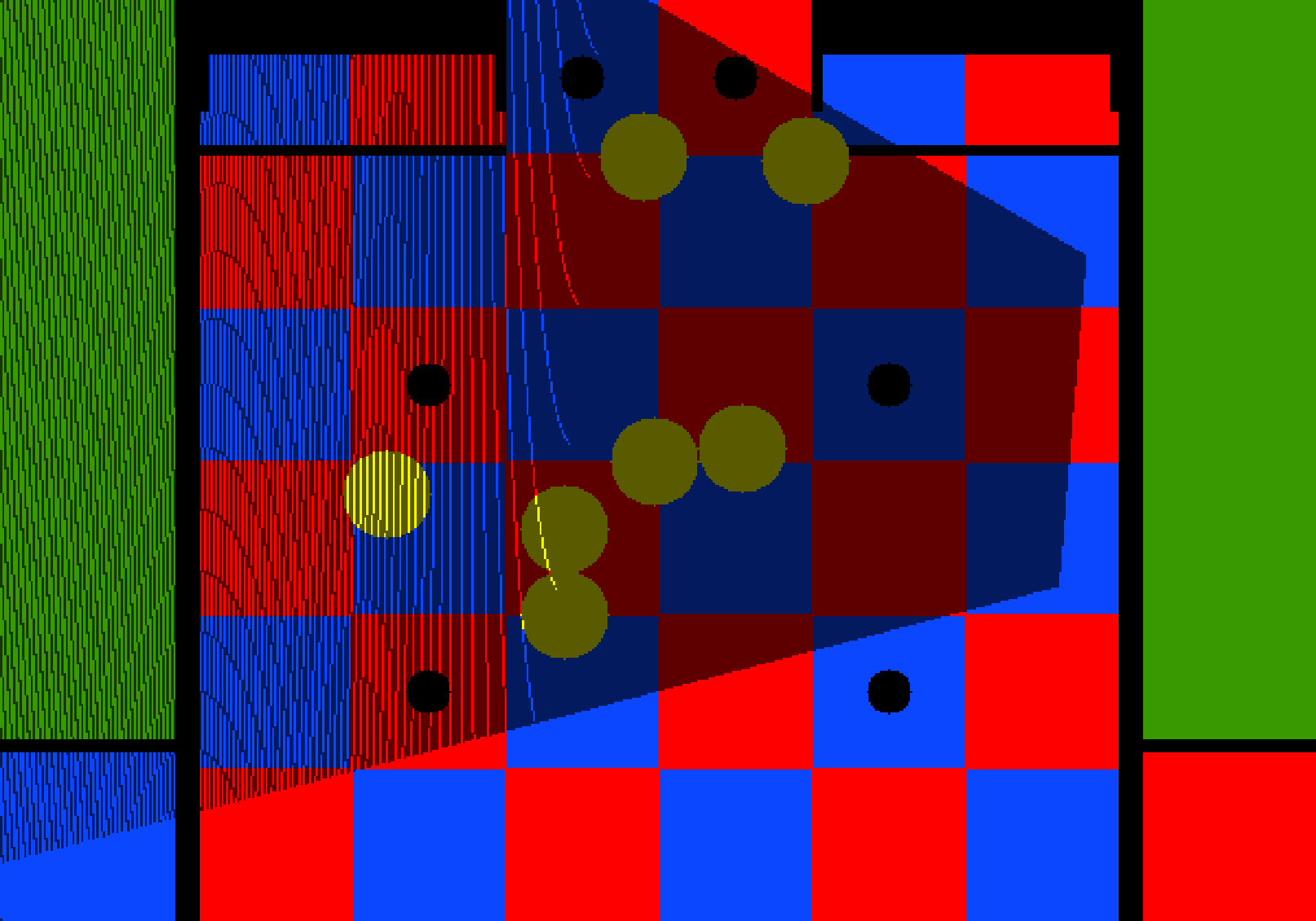## Introduction

Let’s do a summary of what we have done so far:

• COT: colour thresholding. We separated yellow objects from the rest.
• BLED: blob edge detection. We retrieved the bottom edges of blobs (pawns).
• T: transformation. We transformed the image’s pixels into game field points (aka. pixels to meters).

And the last step CID: Circles Detection.

As you may have already noticed, pawns and tower of pawns are in fact circles when viewed from above. Therefore, the bottom edges we found with BLED are also circles' segments when transformed into game field coordinates (step T). This is the property we’re exploiting below.

## Algorithm

The secret here is to find the centre of the circles defined by the transformed edges. Then, we need to remove those which lay outside the game field, and finally we should merge those circles which are too close to each other.

Transform the bottom edges to field coordinates.
For each bottom edge
Retrieve the 2 points that are the farthest from each other (The longer the edge, the more precise the centre is likely to be) :
pLeft  = beginning of the bottom edge
pRight = end of the bottom edge
Given two points and the circle's radius (pLeft, pRight, r), find the circle's centre c

Remove any circle placed outside the game field

If any circle c overlaps any other circle c' then
merge the circles' centre by averaging their positions with the means of a weighted mean to increase precision. The weight being the length of the edge.



Finding a circle’s centre is just a matter of solving $(x - h)^2 + (y - k)^2 = r^2$ for $c=\begin{pmatrix} h \\ k \end{pmatrix}$ given 2 points $p_1=\begin{pmatrix} x_1 \\ y_1 \end{pmatrix}$, $p_2 = \begin{pmatrix} x_2 \\ y_2 \end{pmatrix}$ and the radius $r$.

It can be shown that the centre of the circle is given by: $c = \frac{1}{2}(p_1+p2 )\pm \sqrt{(\frac{r}{d})^2-\frac{1}{4}} \begin{pmatrix} 0 & -1 \\ 1 & 0 \end{pmatrix} (p_2-p_1)$ where $d = ||p_2 - p_1||$

This equation has 2 solutions but only one gives the expected result. To choose the right sign, check whether $p_1$ and $p_2$ go counter-clockwise if yes the the sign should be $+$. In our case we go counter-clockwise because the points are always given from left to right.

## Results

The original image:The binary thresholded image:The bottom edges:The transformed bottom edges with the circles rendered on top of them:## Discussion

We presented a simple yet effective method for finding circles' centres. This is evidently not the only way of finding them, the more usual approach is by using Hough Cirlce Transforms. However, our method is much faster and does not have any special memory requirements as it is not probabilistic and requires only two points, and because the radius is known, high precision is achieved.

Another method we tried was the Least-Squares fit by R. Bullock, which gave pretty much the same results with a sightly heavier computational approach. The advantage of this last method is it uses all points from the edge to compute the circles' centre, this may give better results when the first or the last points of the edge are not correctly found and have an offset. Practically this case rarely happened because pawns, which are yellow, have a very different colour from their entourage which is usually red, blue, green or black, and therefore pawns' bottom edges are usually correctly determined.

## What’s next

The fancy algorithms end here. Yeap, sorry about that. No more maths for today. :)

A conclusion and a summary of what has been done, what could be improved and what I have learned on the next post.